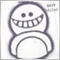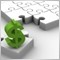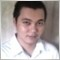How to disable expert trading if it first run340

I made expert which open order if price getting higher or lower value of past day. Backtesting was fine, but problem is when I put expert on Pair, it open order immediately, because current value of price, when I started expert, had greater or lower value than max or low of past day. I need condition: do not start trade if price is greater or lower than extremums of past day and it first expert running on current Pair.8783

Sergey Lapshov:
I made expert which open order if price getting higher or lower value of past day. Backtesting was fine, but problem is when I put expert on Pair, it open order immediately, because current value of price, when I started expert, had greater or lower value than max or low of past day. I need condition: do not start trade if price is greater or lower than extremums of past day and it first expert running on current Pair.
There are a few different ways to do it but it sounds like you have buggy code. Best to post your code up so we can see where the problem is.340

Stuart Browne:
There are a few different ways to do it but it sounds like you have buggy code. Best to post your code up so we can see where the problem is.

I dont know which part of code will be better for post.. I would like to see ways which I can use.

The code is

if(Bid > HighD1+Delta*Point) Open_Bay = true;
if(Bid < LowD1-Delta*Point) Open_Sell = true;

{
if(StopLoss<0){
SL = iLow(NULL,PERIOD_D1,0);
}

if(TakeProfit>0) TP = Ask + TakeProfit*Point;
if(StopLoss>0 ){
SL = Bid - (StopLoss*0.0001);
}
if (Ticket > 0)
{
}
return(0);
}
{
if(TakeProfit>0) TP = Bid - TakeProfit*Point;
if(StopLossValue>StopLoss) SL = Ask + StopLoss ;
if(StopLoss>0 ){SL = Bid + (StopLoss*0.0001); }
Ticket = OrderSend(Symbol(),OP_SELL,GetSizeLot(),Bid,20,SL,TP);
if (Ticket > 0)
{
}
return(0);
}
}
}8783

Sergey Lapshov:

I dont know which part of code will be better for post.. I would like to see ways which I can use.

The code is

Well it's still a bit hard to tell as you have variables in that code that are obviously declared elsewhere( eg HighD1, LowD1 etc). But the most obvious thing to me is your first couple of if statements. If all you're looking for is if the current price is higher/lower than yesterday's high/low, then change the first two ifs to this:

if(Bid > iHigh(Symbol(),PERIOD_D1,1)) Open_Bay = true;
else if(Bid < iLow(Symbol(),PERIOD_D1,1)) Open_Sell = true;

Also, resetting the variable LastTradeTimeBuy to zero at the top of that code negates the later assignment. But again, it's hard to tell what's going on with that snippet of code340

Stuart Browne:

Well it's still a bit hard to tell as you have variables in that code that are obviously declared elsewhere( eg HighD1, LowD1 etc). But the most obvious thing to me is your first couple of if statements. If all you're looking for is if the current price is higher/lower than yesterday's high/low, then change the first two ifs to this:

Also, resetting the variable LastTradeTimeBuy to zero at the top of that code negates the later assignment. But again, it's hard to tell what's going on with that snippet of code

extern double Lots = 0.12;
extern int Delta = 50;
extern int TakeProfit = 600;
extern int StopLoss = 400;
int LastTradeTimeSell = 0;
int LastTradeSell = 0;
double StopLossValue = 0;

int start()
{

double SL=0,TP=0,
StopLevel=Point*MarketInfo(Symbol(),MODE_STOPLEVEL),
HighD1=iHigh(Symbol(),PERIOD_D1,1),
LowD1=iLow(Symbol(),PERIOD_D1,1)
int total = OrdersTotal();
bool Open_Bay=false,Open_Sell=false;
if(Bid > HighD1+Delta*Point) Open_Bay = true;
if(Bid < LowD1-Delta*Point) Open_Sell = true;
int Ticket,cnt,Total=0;
for(cnt=0;cnt<OrdersTotal();cnt++)
{
OrderSelect(cnt,SELECT_BY_POS);
if(OrderSymbol()==Symbol())
{
Total++;

if(Total<2  )
{

{
if(StopLoss<0){
SL = iLow(NULL,PERIOD_D1,0);
}
if(TakeProfit>0) TP = Ask + TakeProfit*Point;
if(StopLoss>0 ){
SL = Bid - (StopLoss*0.0001);
}
if (Ticket > 0)
{
}
return(0);
}
{
if(TakeProfit>0) TP = Bid - TakeProfit*Point;
if(StopLossValue>StopLoss) SL = Ask + StopLoss ;
if(StopLoss>0 ){SL = Bid + (StopLoss*0.0001); }
Ticket = OrderSend(Symbol(),OP_SELL,Lots,Bid,20,SL,TP);
if (Ticket > 0)
{
}
return(0);
}
}
}7044

I think what you wanted is for the EA to wait for the end of the current bar before it starts to enter trades. Detecting the formation of a new bar on the EA will help in this case.340

Enrico Lambino:
I think what you wanted is for the EA to wait for the end of the current bar before it starts to enter trades. Detecting the formation of a new bar on the EA will help in this case.

Here is solution

double fPreviousBid = 0;

int OnInit()
{
fPreviousBid = 0;
return(INIT_SUCCEEDED);
}

void OnTick()
{

double fUpperLevel = iHigh(Symbol(), PERIOD_D1, 1) + Delta * Point();
double fLowerLevel = iLow(Symbol(), PERIOD_D1, 1) - Delta * Point();

bool bBuySignal = fPreviousBid > 0 && Bid > fUpperLevel && fPreviousBid <= fUpperLevel;
bool bSellSignal = fPreviousBid > 0 && Bid < fLowerLevel && fPreviousBid >= fLowerLevel;

fPreviousBid = Bid;